JMSLTM Numerical Library 7.2.0
com.imsl.math

Class CsSmoothC2

• All Implemented Interfaces:
Serializable, Cloneable

public class CsSmoothC2
extends Spline
Extension of the Spline class used to construct a spline for noisy data points using an alternate method.

Class CsSmoothC2 is designed to produce a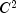cubic spline approximation to a data set in which the function values are noisy. This spline is called a smoothing spline. It is a natural cubic spline with knots at all the data abscissas x, but it does not interpolate the data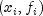. The smoothing spline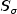is the uniquefunction that minimizes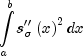subject to the constraint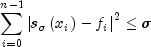.

Recommended values fordepend on the weights, w. If an estimate for the standard deviation of the error in the y-values is availiable, then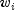should be set to this value and the smoothing parameter should be choosen in the confidence interval corresponding to the left side of the above inequality. That is,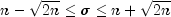CsSmoothC2 is based on an algorithm of Reinsch (1967). This algorithm is also discussed in de Boor (1978, pages 235-243).
Example, Serialized Form
• Constructor Detail

• CsSmoothC2

public CsSmoothC2(double[] xData,
double[] yData,
double sigma)
Constructs a smooth cubic spline from noisy data using an algorithm based on Reinsch (1967). All of the points have equal weights.
Parameters:
xData - A double array containing the x-coordinates of the data. Values must be distinct.
yData - A double array containing the y-coordinates of the data. The arrays xData and yData must have the same length.
sigma - A double value specifying the smoothing parameter. Sigma must not be negative.
• CsSmoothC2

public CsSmoothC2(double[] xData,
double[] yData,
double[] weight,
double sigma)
Constructs a smooth cubic spline from noisy data using an algorithm based on Reinsch (1967) with weights supplied by the user.
Parameters:
xData - A double array containing the x-coordinates of the data. Values must be distinct.
yData - A double array containing the y-coordinates of the data. The arrays xData and yData must have the same length.
weight - A double array containing the weights. The arrays xData and weight must have the same length.
sigma - A double value specifying the smoothing parameter. Sigma must not be negative.
JMSLTM Numerical Library 7.2.0